Function Repository Resource:

# FitPowerLaw

Fit a power law to data

Contributed by: Sander Huisman
 ResourceFunction["FitPowerLaw"][data] fits data with a power law by fitting the log of data with a linear function. ResourceFunction["FitPowerLaw"][data,x] fits data and uses the variable x for the resulting fits.

## Details and Options

ResourceFunction["FitPowerLaw"] effectively fits the function a xb to the data, but does so by fitting the linear function c x+d to the log of the data.
ResourceFunction["FitPowerLaw"] fits in 'log space' such that fits that span multiple decades of data are not dominated by a few of the largest values and the smallest values are basically neglegible. The errors are therefore not in the sense of a classical least-square but rather the square of the log of the ratio of the fitted value and the original data. Visually this then results in a 'good fit' when the data is visualized in a log-log plot of the data.
The data data should either be a list of points {y1, y2, y3, …,yn} (to which the x coordinates 1 through n are assigned), or the data is {x,y} pairs: {{x1,y1},{x2,y2},…}, for a list of lists of data ResourceFunction["FitPowerLaw"] will fit each sublist, returning in that case a list of associations.
Only positive values are fitted. Negative points are ignored.
ResourceFunction["FitPowerLaw"] has the following options:
 "Exponent" Automatic prescribe the exponent "Prefactor" Automatic prescribe the prefactor
If both options are given, only the "Prefactor" option is used.
ResourceFunction["FitPowerLaw"] returns an Association with fit and fit parameters.

## Examples

### Basic Examples (1)

Create some data and fit it:

 In:=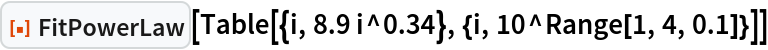Out=### Scope (3)

For a single list of values the x coordinates are assumed to be 1,2,3,4…:

 In:=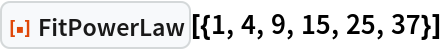Out=In:=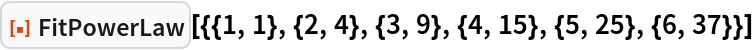Out=For a list of lists of data each list will be fitted separately:

 In:=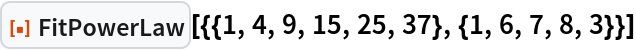Out=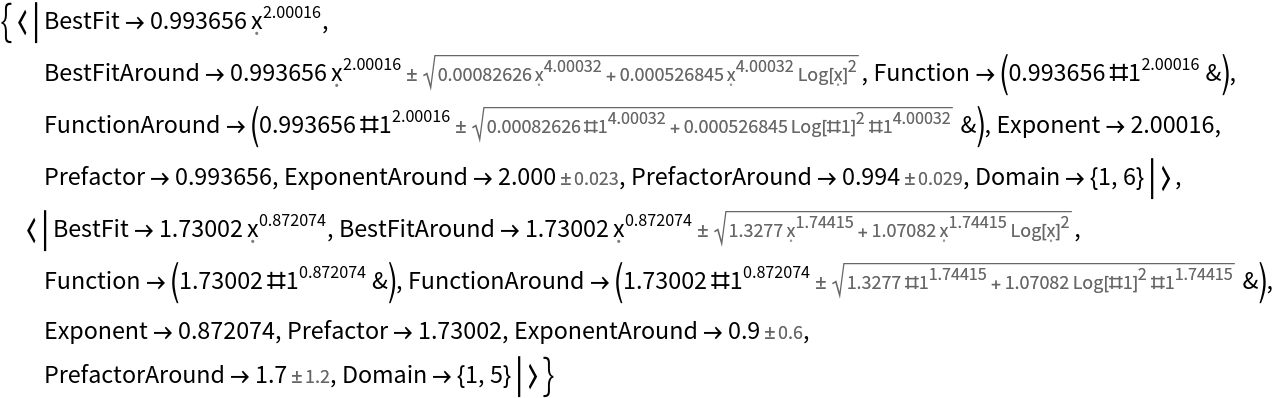For a list of pairs the data is assumed to be in {x,y} pairs:

 In:=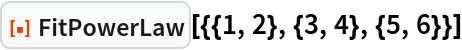Out=### Options (2)

Prescribe the exponent:

 In:=Out=Prescribe the prefactor:

 In:=Out=### Applications (1)

Create some data, fit it, and then plot both:

 In:=Out=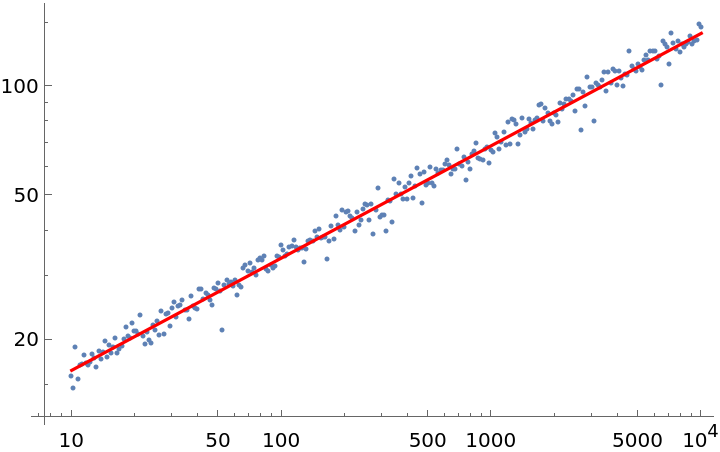### Possible Issues (3)

The difference between a classical least-squares fit or the fitting of the least-squares in log-space becomes very apparent when fitting over several decades. Because the error is much larger for the higher decades the error is completely dominated by those larger points, resulting in a strange fit when viewed in log-space:

 In:=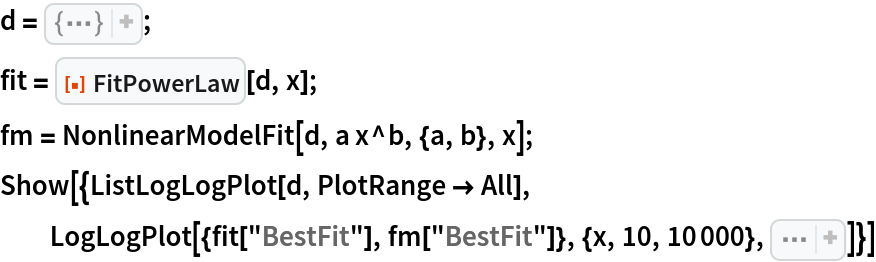Out=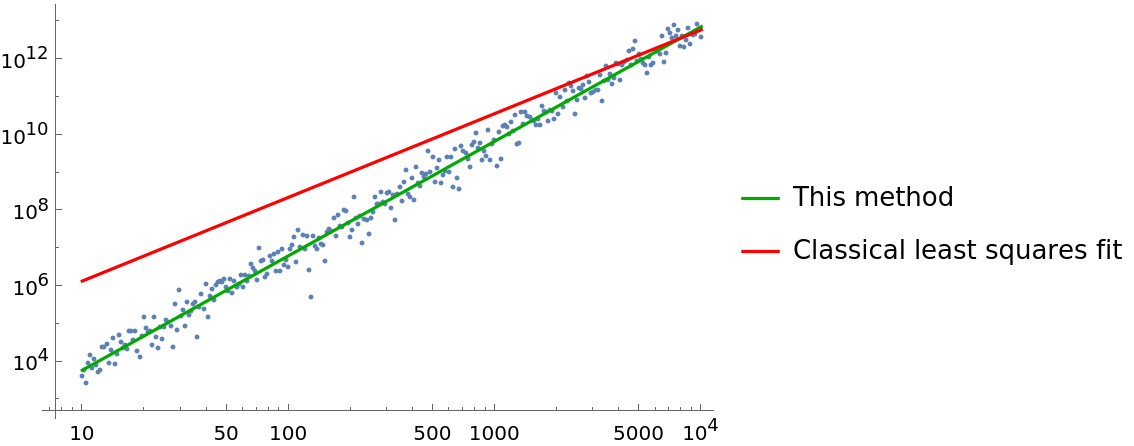The error for the classical method is lower:

 In:=Out=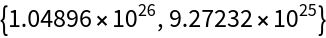But not in log space, here the current method is lower:

 In:=Out=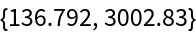SHuisman

## Version History

• 1.0.0 – 07 October 2022